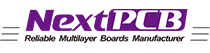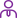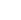### New Life with Your NEXTPCB!

Turnkey PCB manufacturing & assembly services.

LanguageLogin|Sign up
Blog >> Blog Details Page
Inductance Analysis of Through Holes in Printed Circuit Boards
Posted:05:20 PM July 30, 2018 writer: G

The purpose of the bypass capacitor is to short the two power planes together at high frequencies. If you assume that an integrated circuit is connected between power and ground plane at point a, there is an ideal surface mount bypass capacitor at point b. It is expected that the high frequency impedance between the vcs of the die pad and the ground plane is zero. However, this is not the case. Each connection via inductance that connects the capacitor to vcc and ground plane introduces a small but measurable inductance. The size of this inductor is approximately:

Where l = through hole inductance, nh

h=through hole length, in

d = through hole diameter, in

Since the above equation includes a logarithm, the change in the diameter of the via has little effect on the inductance, but the change in the length of the via may cause a large change.

The through hole is inductive to a signal with a rising edge speed of 1 ns. First calculate the inductance:

h=0.063 (through hole length, in)

d=0.016 (through hole diameter, in)

T10~90%=1.00 (rising edge speed, ns)

The high-frequency current is split from the chip, and the value of 3.8 ohms is not low enough. Also keep in mind that the bypass capacitor is usually connected to the ground plane through one via at one end and to the +5v plane through a via at the other end, so the effect of the via inductance is doubled. The bypass capacitor is placed on the side of the board closest to the power and ground planes to help reduce its effects. Finally, any leads between the capacitor and the via will add more inductance. These lines should always be as wide as possible.

Using multiple bypass capacitors between the power supply and ground provides very low impedance. For digital products, as a rough guideline, assume that the power supply and ground plane are ideal conductors with zero inductance. We only consider the inductance of the bypass capacitor and its associated traces and vias. Within a specific range, all bypass capacitors will act in parallel, reducing the impedance between the power supply and ground. The effective radius at which this effect is produced is equal to 1/12, where 1 is the electrical length of the rising edge. Within 1/6 of the diameter, all capacitors together act as a lumped circuit.

The 1 ns rising edge has a propagation length of approximately 1 = 6 in the fr-4 material. In this example, the grid spacing of the capacitors is greater than 1/12 = 0.5 in, and there will be no benefit.

For bypass capacitors of the power supply, the shorter the rise time, the more difficult the bypass becomes. When the rise time is shortened, the value of the effective radius also becomes smaller. The amount of capacitance within the effective radius decreases with the square of the rise time.

This is a comprehensive question. As the rise time decreases, the digital corner frequency increases, increasing the inductive reactance of each via. The net result is that for a specific configuration of bypass capacitors operating at a certain frequency, when we halve the rise time, the effect is reduced by a factor of eight. Based on this scaling criterion, experience gained from a range of operating frequencies can be easily converted to a new operating frequency range.

501 0 0 1
• PCB
Portotype
• PCB
Assembly
• SMD
Stencil
 Dimensions: (mm) × Quantity: (pcs) 5 5 10 15 20 25 30 40 50 75 100 120 150 200 250 300 350 400 450 500 600 700 800 900 1000 1500 2000 2500 3000 3500 4000 4500 5000 5500 6000 6500 7000 7500 8000 9000 10000 Other Quantities:(quantity*length*width is greater than 10㎡) OK Layers: 1 2 4 6 8 10 12 Thickness: 0.6 mm 0.8 mm 1.0 mm 1.2 mm 1.6 mm 2.0 mm 2.5 mmQuote now